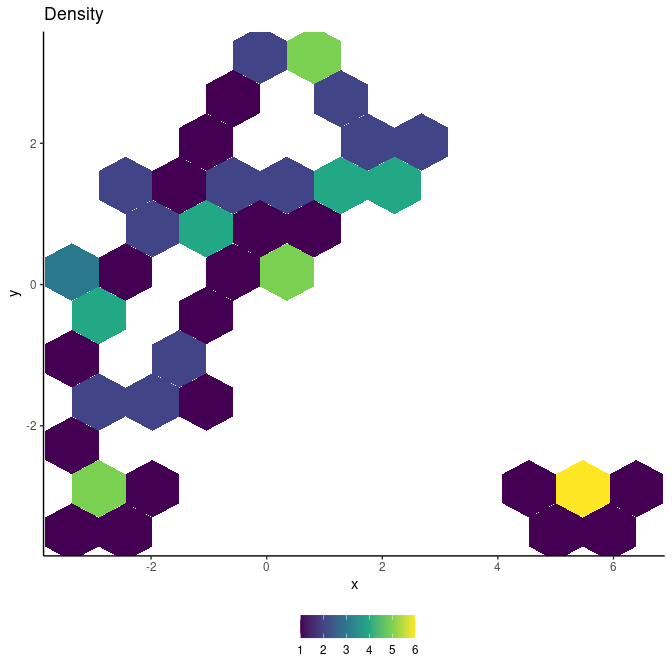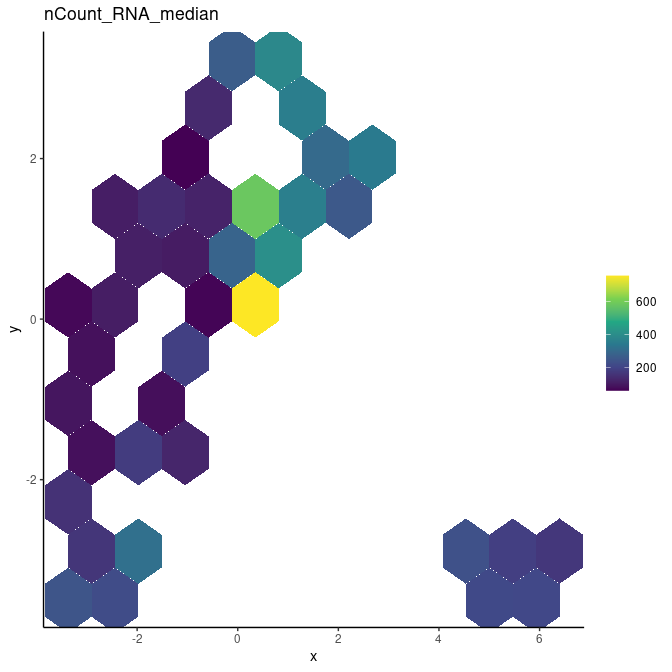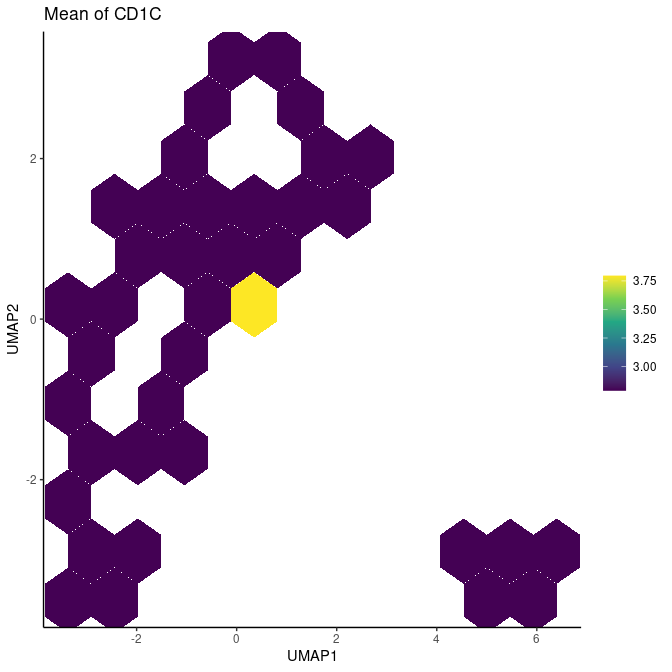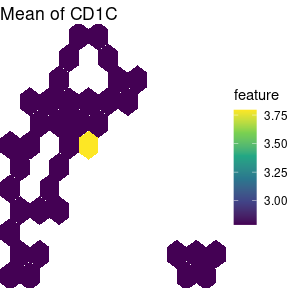Using schex with Seurat

Reduced dimension plotting is one of the essential tools for the analysis of single cell data. However, as the number of cells/nuclei in these plots increases, the usefulness of these plots decreases. Many cells are plotted on top of each other obscuring information, even when taking advantage of transparency settings. This package provides binning strategies of cells/nuclei into hexagon cells. Plotting summarized information of all cells/nuclei in their respective hexagon cells presents information without obstructions. The package seemlessly works with the two most common object classes for the storage of single cell data; SingleCellExperiment from the SingleCellExperiment package and Seurat from the Seurat package. In this vignette I will be presenting the use of schex for Seurat objects.

library(schex)
library(dplyr)
library(Seurat)

Setup single cell data

In order to demonstrate the capabilities of the schex package, I will use the a subsetted dataset of Peripheral Blood Mononuclear Cells (PBMC) freely available from 10x Genomics. There are 80 single cells with 230 features in this dataset.

pbmc_small
#> An object of class Seurat
#> 230 features across 80 samples within 1 assay
#> Active assay: RNA (230 features, 20 variable features)
#>  2 dimensional reductions calculated: pca, tsne

Perform dimensionality reductions

The dataset already contains two dimension reductions (PCA and TSNE). We will now add UMAP. Since there is a random component in the UMAP, we will set a seed.

Plotting single cell data

At this stage in the workflow we usually would like to plot aspects of our data in one of the reduced dimension representations. Instead of plotting this in an ordinary fashion, I will demonstrate how schex can provide a better way of plotting this.

Calculate hexagon cell representation

First, I will calculate the hexagon cell representation for each cell for a specified dimension reduction representation. I decide to use nbins=40 which specifies that I divide my x range into 10 bins. Note that this might be a parameter that you want to play around with depending on the number of cells/ nuclei in your dataset. Generally, for more cells/nuclei, nbins should be increased.

Plot number of cells/nuclei in each hexagon cell

First I plot how many cells are in each hexagon cell. This should be relatively even, otherwise change the nbins parameter in the previous calculation.Plot meta data in hexagon cell representation

Next I colour the hexagon cells by some meta information, such as the median total count in each hexagon cell.Plot gene expression in hexagon cell representation

Finally, I will visualize the gene expression of the CD1C gene in the hexagon cell representation.Understanding schex output as ggplot objects

The schex packages renders ordinary ggplot objects and thus these can be treated and manipulated using the ggplot grammar. For example the non-data components of the plots can be changed using the function theme.The fact that schex renders ggplot objects can also be used to save these plots. Simply use ggsave in order to save any created plot.